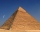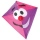# Pythagorean theorem - 8th grade (13y) - math problems

#### Number of problems found: 412

• Triangular pyramidCalculate the volume of a regular triangular pyramid with edge length a = 12cm and pyramid height v = 20cm.
• The regularThe regular triangular prism has a base in the shape of an isosceles triangle with a base of 86 mm and 6.4 cm arms, the height of the prism is 24 cm. Calculate its volume.
• Hexagonal pyramidFind the area of a shell of the regular hexagonal pyramid, if you know that its base edge is 5 cm long and the height of this pyramid is 10 cm.
• The pyramidThe pyramid with a square base is 50 m high and the height of the sidewall is 80 m. Find the endge of the base of the pyramid.
• Surface of the coneCalculate the surface of the cone if its height is 8 cm and the volume is 301.44 cm3.
• Sphere parts, segmentA sphere with a diameter of 20.6 cm, the cut is a circle with a diameter of 16.2 cm. .What are the volume of the segment and the surface of the segment?A quadrilateral pyramid, which has a rectangular base with dimensions of 24 cm, 13 cm. The height of the pyramid is 18cm. Calculate 1/the area of the base 2/casing area 3/pyramid surface 4/volume of the pyramid
• Two parallel chordsIn a circle 70 cm in diameter, two parallel chords are drawn so that the center of the circle lies between the chords. Calculate the distance of these chords if one of them is 42 cm long and the second 56 cm.
• Height of pyramidThe pyramid ABCDV has edge lengths: AB = 4, AV = 7. What is its height?
• SailboatThe 20 m long sailboat has an 8 m high mast in the middle of the deck. The top of the mast is fixed to the bow and stern with a steel cable. Determine how much cable is needed to secure the mast and what angle the cable will make with the ship's deck.
• Railway embankmentThe section of the railway embankment is an isosceles trapezoid, the sizes of the bases of which are in the ratio 5: 3. The arms have a length of 5 m and the height of the embankment is 4.8 m. Calculates the size of the embankment section area.
• Base of an isosceles triangleCalculate the size of the base of an isosceles triangle, the height is 5 cm and the length of the arm is 6.5 cm. What is the perimeter of this triangle?
• Two chordsIn a circle with radius r = 26 cm two parallel chords are drawn. One chord has a length t1 = 48 cm and the second has a length t2 = 20 cm, with the center lying between them. Calculate the distance of two chords.
• A kiteChildren have a kite on an 80m long rope, which floats above a place 25m from the place where children stand. How high is the dragon floating above the terrain?
• Rectangular base pyramidCalculate an area of the shell of the pyramid with a rectangular base of 2.8 m and 1.4 m and height 2.5 meters.
• How manyHow many m2 of copper sheet is needed to replace the roof of a conical tower with a diameter of 13 meters and a height of 24 meters, if we count 8% of the material for bending and waste?
• Right angleIn a right triangle ABC with a right angle at the apex C, we know the side length AB = 24 cm and the angle at the vertex B = 71°. Calculate the length of the legs of the triangle.Adam placed the ladder of the house, the upper end reaching to the window at the height of 3.6m, and the lower end standing on level ground and was distant from a wall of 1.5m. What is the length of the ladder?
• Median in right triangleIn the rectangular triangle ABC has known the length of the legs a = 15cm and b = 36cm. Calculate the length of the median to side c (to hypotenuse).
• Truncated cone 6Calculate the volume of the truncated cone whose bases consist of an inscribed circle and a circle circumscribed to the opposite sides of the cube with the edge length a=1.

Do you have an interesting mathematical word problem that you can't solve it? Submit a math problem, and we can try to solve it.

We will send a solution to your e-mail address. Solved examples are also published here. Please enter the e-mail correctly and check whether you don't have a full mailbox.

Please do not submit problems from current active competitions such as Mathematical Olympiad, correspondence seminars etc...

Pythagorean theorem is the base for the right triangle calculator.